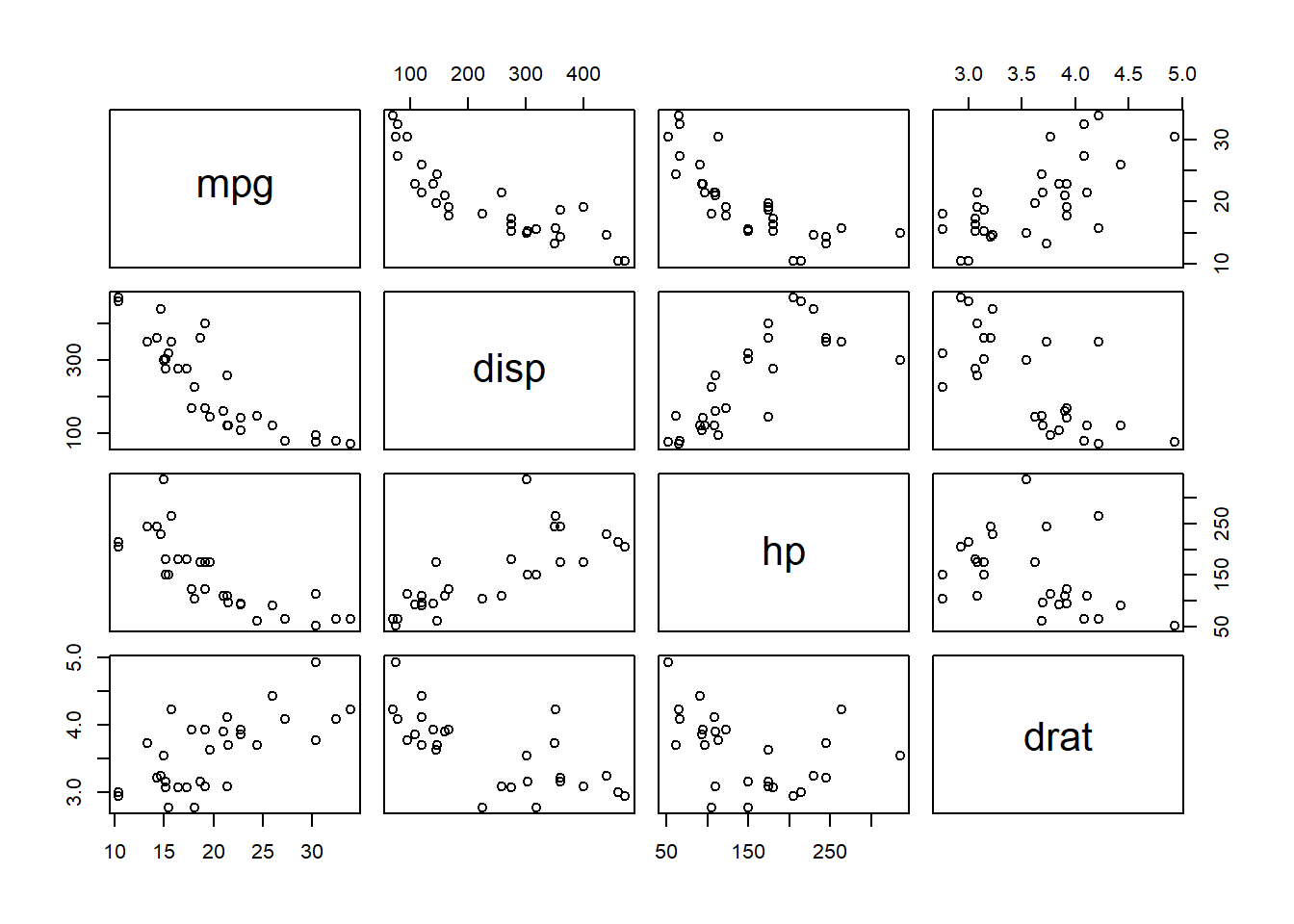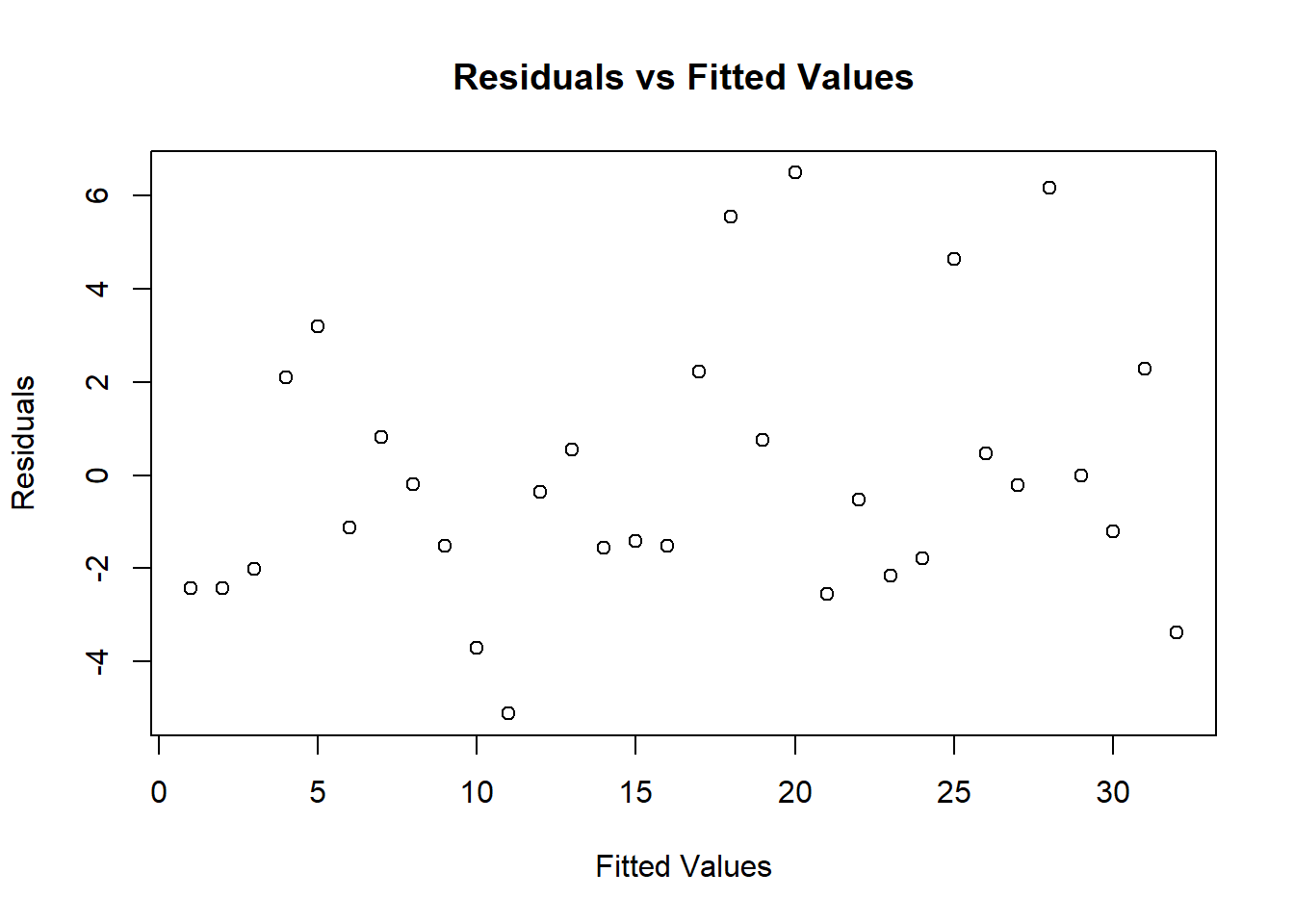# How to Perform Multiple Linear Regression in R

rtip
Author

Steven P. Sanderson II, MPH

Published

November 15, 2023

# Introduction

Multiple linear regression is a powerful statistical method that allows us to examine the relationship between a dependent variable and multiple independent variables.

# Example

## Step 1: Load the dataset

``````# Load the mtcars dataset
data(mtcars)``````

## Step 2: Build the model

Now, let’s create the multiple linear regression model using the specified variables: disp, hp, and drat.

``````# Build the multiple linear regression model
model <- lm(mpg ~ disp + hp + drat, data = mtcars)``````

## Step 3: Examine the data

It’s always a good idea to take a look at the relationships between variables before diving into the model. The `pairs()` function helps us with that.

``````# Examine relationships between variables
pairs(mtcars[,c("mpg","disp","hp","drat")])``````## Step 4: Check for multicollinearity

Multicollinearity is when independent variables in a regression model are highly correlated. It can affect the stability and reliability of our model. Keep an eye on the scatterplots in the pairs plot to get a sense of this.

## Step 5: Plot the residuals

Now, let’s check the model’s residuals using a scatterplot. Residuals are the differences between observed and predicted values. They should ideally show no pattern.

``````# Plot the residuals
plot(
model\$residuals,
main = "Residuals vs Fitted Values",
xlab = "Fitted Values",
ylab = "Residuals"
)``````## Step 6: Evaluate the model

By examining the residuals vs. fitted values plot, we can identify patterns that may suggest non-linearity or heteroscedasticity. Ideally, residuals should be randomly scattered.

## Step 7: Encourage readers to try it themselves

I’d encourage readers to take the code snippets, run them in their R environment, and explore. Maybe try different variables, tweak the model, or even use another dataset. Hands-on experience is the best teacher!

Remember, understanding the data and interpreting the results is as important as running the code. It’s a fascinating journey into uncovering patterns and relationships within your data.

Feel free to reach out if you have any questions or if there’s anything specific you’d like to explore further. Happy coding!